### Type 3 Tests

The following statistics can be used to test the null hypothesis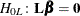, whereis a matrix of known coefficients. Under mild assumptions, each of the following statistics has an asymptotic chi-square distribution with
degrees of freedom, where p is the rank of. Let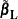be the maximum likelihood ofunder the null hypothesis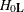; that is,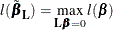#### Likelihood Ratio Statistic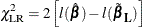#### Score Statistic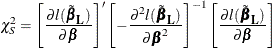#### Wald’s Statistic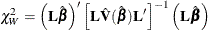where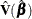is the estimated covariance matrix, which can be the model-based covariance matrix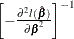or the sandwich covariance matrix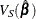(see the section Robust Sandwich Variance Estimate for details).